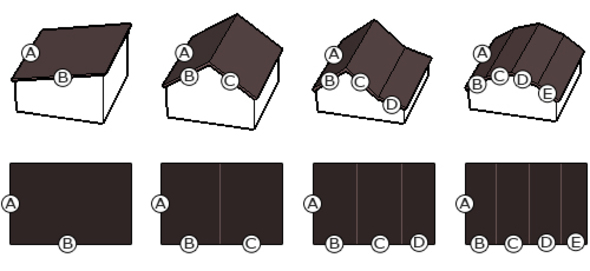20427
Math

# Field parabola

The calculator calculates the surface area of ​​the parabola with the given base width and length of the perpendicular [see calculator squareness]. The result is given as the user input unit square.

see similar
1260344
Math

## Weighted average ratings

The calculator calculates the weighted average of the ratings of the following items.
258207
Math

## Conversion between number bases

Converter allows you to convert numbers between number bases - binary (binary), trójkowym, czwórkowym, Friday, szóstkowym, siódemkowym, octal (octal), dziewiątkowym, decimal (decymalnym) jedenastkowym, dwunastkowym, trzynastkowym, czternastkowym, piętnastkowym, hexadecimal (hex). Typed characters that are not numbers and the letters A, B, C, D, E, F are ignored.
123572
Math

## Trigonometric functions calculator

Calculator by entering an angle in degrees or radians calculated values ​​of the basic trigonometric functions.
Users also viewed
3311255
Building

## Calculator floor panels

Calculator based on the dimensions of the area to put the panels and occupancy data on number of panels in one package, calculate how much you have to buy packages of panels to cover the entire room.
213108
Building

## Kalkulator powierzchni dachu

Jak obliczyć powierzchnię dachu?1466130
Life

## Rainwater tank

The calculator calculates the amount of collected rainwater from the roof of the given dimensions. Long description
80871
Health

## BMR Calculator - Basic Metabolic - PPM

Jak obliczyć wskaźnik BMR / PPM ?
The calculator calculates the BMR (Basal Metabolic Rate). BMR - the lowest rate of metabolism that occur in the human body, necessary to sustain basic life functions, which is located in standby mode under complete peace of physical and mental comfort that the 12 hours before the test, do not eat any meals, after 3 days of diet and protein-free after at least eight hours of sleep.
25544
Math

## Field and circumference of a circle

Jak obliczyć pole i obwód koła?
The calculator based on the specified radius of the area and perimeter of a circle.